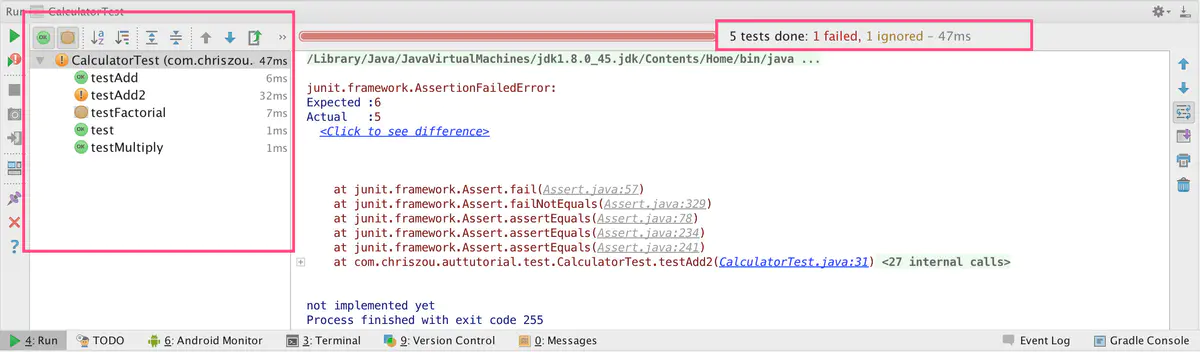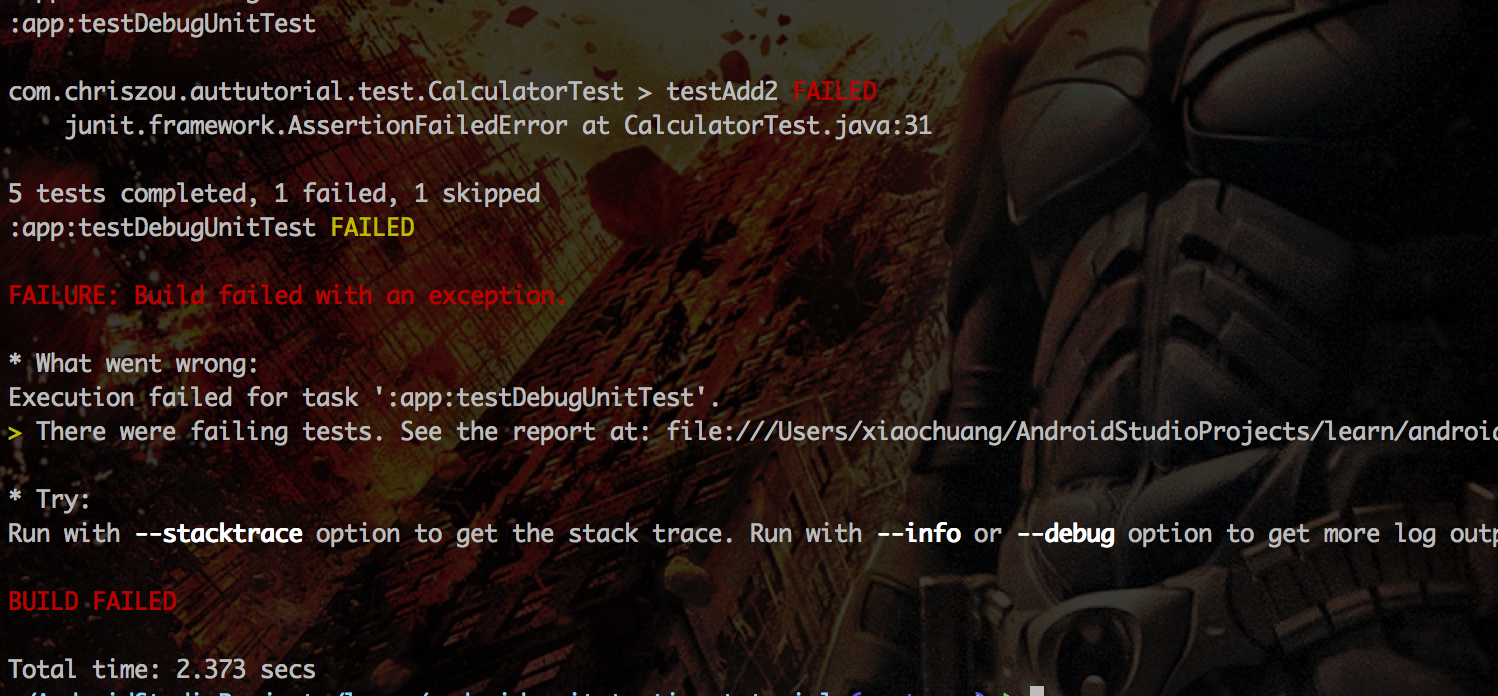## 为什么要使用单元测试框架

``````public class Calculator {
public int add(int one, int another) {
// 为了简单起见，暂不考虑溢出等情况。
return one + another;
}

public int multiply(int one, int another) {
// 为了简单起见，暂不考虑溢出等情况。
return one * another;
}
}``````

``````public class CalculatorTest {
public static void main(String[] args) {
Calculator calculator = new Calculator();
if(sum == 3) {
} else {
}

int product = calculator.multiply(2, 4);
if (product == 8) {
System.out.println("multiply() works!")
} else {
System.out.println("multiply() does not works!")
}
}
}``````

``````public class CalculatorTest {

@Test
public void testAdd() throws Exception {
Calculator calculator = new Calculator();
Assert.assertEquals(3, sum);
}

@Test
public void testMultiply() throws Exception {
Calculator calculator = new Calculator();
int product = calculator.multiply(2, 4);
Assert.assertEquals(8, product);
}

}``````

1. setup
2. 执行操作
3. 验证结果

``````public class CalculatorTest {
Calculator mCalculator;

@Before
public void setup() {
mCalculator = new Calculator();
}

@Test
public void testAdd() throws Exception {
assertEquals(3, sum);  //为了简洁，往往会static import Assert里面的所有方法。
}

@Test
public void testMultiply() throws Exception {
int product = mCalculator.multiply(2, 4);
assertEquals(8, product);
}

}``````

`assertEquals(expected, actual)`

`assertEquals(expected, actual, tolerance)`

`assertTrue(boolean condition)`

`assertFalse(boolean condition)`

`assertNull(Object obj)`

`assertNotNull(Object obj)`

`assertSame(expected, actual)`

`assertNotSame(expected, actual)`

`fail()`

`assertEquals("Current user Id should be 1", 1, currentUser.id());`
`currentUser.id()`的值不是 1 的时候，在结果报道里面将显示"Current user Id should be 1"，这样可以让测试结果更具有可读性，更清楚错误的原因是什么。

## JUnit 的其他功能

#### Ignore 一些测试方法

``````public class CalculatorTest {
Calculator mCalculator;

@Before
public void setup() {
mCalculator = new Calculator();
}

// Omit testAdd() and testMultiply() for brevity

@Test
@Ignore("not implemented yet")
public void testFactorial() {
}
}``````

#### 验证方法会抛出某些异常

``````public class Calculator {

// Omit testAdd() and testMultiply() for brevity

public double divide(double divident, double dividor) {
if (dividor == 0) throw new IllegalArgumentException("Dividor cannot be 0");

return divident / dividor;
}}``````

``````public class CalculatorTest {
Calculator mCalculator;

@Before
public void setup() {
mCalculator = new Calculator();
}

// Omit testAdd() and testMultiply() for brevity

@Test(expected = IllegalArgumentException.class)
public void test() {
mCalculator.divide(4, 0);
}

}``````

`@Test(expected = IllegalArgumentException.class)`表示验证这个测试方法将抛出`IllegalArgumentException`异常，如果没有抛出的话，则测试失败。

## 在 Android 项目里面使用 JUnit

`testCompile 'junit:junit:4.12'`## 小结